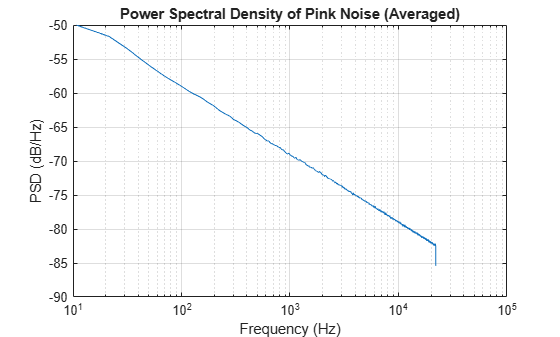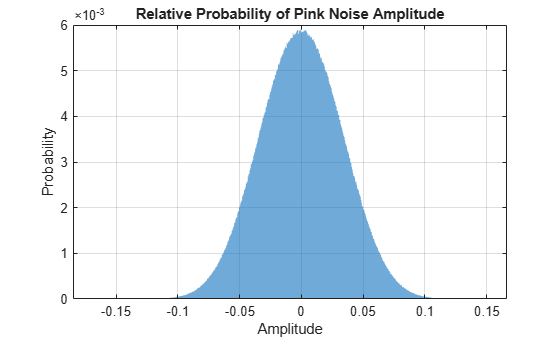# pinknoise

Generate pink noise

## Syntax

``X = pinknoise(n)``
``X = pinknoise(sz1,sz2)``
``X = pinknoise(sz)``
``X = pinknoise(___,typename)``
``X = pinknoise(___,'like',p)``

## Description

example

````X = pinknoise(n)` returns a pink noise column vector of length `n`.```

example

````X = pinknoise(sz1,sz2)` returns a `sz1`-by-`sz2` matrix. Each channel (column) of the output `X` is an independent pink noise signal.```

example

````X = pinknoise(sz)` returns a vector or matrix with dimensions defined by the elements of vector `sz`. `sz` must be a one- or two-element row vector of positive integers. Each channel (column) of the output `X` is an independent pink noise signal.```
````X = pinknoise(___,typename)` returns an array of pink noise of data type `typename`. The `typename` input can be either `'single'` or `'double'`. You can combine `typename` with any of the input arguments in the previous syntaxes.```

example

````X = pinknoise(___,'like',p)` returns an array of pink noise like `p`. You can specify either `typename` or `'like'`, but not both.```

## Examples

collapse all

Generate 100 seconds of pink noise with a sample rate of 44.1 kHz.

```fs = 44.1e3; duration = 100; y = pinknoise(duration*fs);```

Plot the average power spectral density (PSD) of the generated pink noise.

```[~,freqVec,~,psd] = spectrogram(y,round(0.05*fs),[],[],fs); meanPSD = mean(psd,2); semilogx(freqVec,db(meanPSD,"power")) xlabel('Frequency (Hz)') ylabel('PSD (dB/Hz)') title('Power Spectral Density of Pink Noise (Averaged)') grid on```Generate 500 seconds of pink noise with a sample rate of 16 kHz.

```fs = 16e3; duration = 500; y = pinknoise(duration*fs);```

Plot the relative probability of the pink noise amplitude. The amplitude is always bounded between $-$1 and 1.

```histogram(y,"Normalization","probability","EdgeColor","none") xlabel("Amplitude") ylabel("Probability") title("Relative Probability of Pink Noise Amplitude") grid on```Create a 5 second stereo pink noise signal with a 48 kHz sample rate.

```fs = 48e3; duration = 5; numChan = 2; pn = pinknoise(duration*fs,numChan);```

Listen to the stereo pink noise signal.

`sound(pn,fs)`

Channels of the pink noise function are generated independently. Note that the off-diagonal correlation coefficients are close to zero (uncorrelated).

`R = corrcoef(pn(:,1),pn(:,2))`
```R = 2×2 1.0000 -0.0190 -0.0190 1.0000 ```

Correlated and uncorrelated pink noise have different psychoacoustic effects. When the noise is correlated, the sound is less ambient and more centralized. To listen to correlated pink noise, send a single channel of the pink noise signal to your stereo device. The effect is most pronounced when using headphones.

`sound([pn(:,1),pn(:,1)],fs)`

`[audioIn,fs] = audioread("MainStreetOne-24-96-stereo-63secs.wav");`

Create a pink noise signal of the same size and data type as `audioIn`.

`noise = pinknoise(size(audioIn),'like',audioIn);`

Add the pink noise to the audio signal and then listen to the first 5 seconds.

```noisyMainStreet = noise + audioIn; sound(noisyMainStreet(1:fs*5,:),fs)```

The `pinknoise` function generates an approximate $-$29.5 dB signal level, which is close to the power of the audio signal.

```noisePower = sum(noise.^2,1)/size(noise,1); signalPower = sum(audioIn.^2,1)/size(audioIn,1); snr = 10*log10(signalPower./noisePower)```
```snr = 1×2 -0.3505 -1.6718 ```
`noisePowerdB = 10*log10(noisePower)`
```noisePowerdB = 1×2 -29.6072 -29.5546 ```
`signalPowerdB = 10*log10(signalPower)`
```signalPowerdB = 1×2 -29.9577 -31.2264 ```

Mix the input audio with the generated pink noise at an 8 dB SNR.

```desiredSNR = 8; scaleFactor = sqrt(signalPower./(noisePower*(10^(desiredSNR/10)))); noise = noise.*scaleFactor;```

Verify the resulting SNR is 8 dB and then listen to the first 5 seconds.

```noisePower = sum(noise.^2,1)/size(noise,1); snr = 10*log10(signalPower./noisePower)```
```snr = 1×2 8.0000 8.0000 ```
```noisyMainStreet = noise + audioIn; sound(noisyMainStreet(1:fs*5,:),fs)```

## Input Arguments

collapse all

Number of rows of pink noise, specified as a nonnegative integer.

Data Types: `single` | `double` | `int8` | `int16` | `int32` | `int64` | `uint8` | `uint16` | `uint32` | `uint64`

Size of each dimension, specified as a nonnegative integer or two separate arguments of nonnegative integers.

Data Types: `single` | `double` | `int8` | `int16` | `int32` | `int64` | `uint8` | `uint16` | `uint32` | `uint64`

Size of each dimension, specified as a one- or two-element row vector of nonnegative integers. Each element of this vector indicates the size of the corresponding dimension.

Data Types: `single` | `double` | `int8` | `int16` | `int32` | `int64` | `uint8` | `uint16` | `uint32` | `uint64`

Data type to create, specified as `'double'` or `'single'`.

Data Types: `char` | `string`

Prototype of array to create, specified as a numeric array. The generated pink noise is the same data type as `p`.

Data Types: `single` | `double`

## Output Arguments

collapse all

Pink noise, returned as a column vector or matrix of independent channels.

Data Types: `single` | `double`

## Algorithms

Pink noise is generated by passing uniformly distributed random numbers through a series of randomly initiated SOS filters. The resulting pink noise amplitude distribution is quasi-Gaussian and bounded between −1 and 1. The resulting pink noise power spectral density (PSD) is inversely proportional to frequency:

`$S\left(f\right)\propto \frac{1}{f}$`

## Extended Capabilities

### C/C++ Code GenerationGenerate C and C++ code using MATLAB® Coder™.